SBAS660C August   2016  – June 2017

PRODUCTION DATA.

1. Features
2. Applications
3. Description
1.     Device Images
4. Revision History
5. Device Family Comparison Table
6. Pin Configuration and Functions
7. Specifications
8. Parameter Measurement Information
9. Detailed Description
1. 9.1 Overview
2. 9.2 Functional Block Diagram
3. 9.3 Feature Description
1. 9.3.1  Multiplexer
2. 9.3.2  Low-Noise Programmable Gain Amplifier
3. 9.3.3  Voltage Reference
4. 9.3.4  Clock Source
5. 9.3.5  Delta-Sigma Modulator
6. 9.3.6  Digital Filter
7. 9.3.7  Excitation Current Sources (IDACs)
8. 9.3.8  Bias Voltage Generation
9. 9.3.9  System Monitor
10. 9.3.10 Status Register
11. 9.3.11 General-Purpose Inputs and Outputs (GPIOs)
12. 9.3.12 Low-Side Power Switch
13. 9.3.13 Cyclic Redundancy Check (CRC)
14. 9.3.14 Calibration
4. 9.4 Device Functional Modes
1. 9.4.1 Reset
2. 9.4.2 Power-Down Mode
3. 9.4.3 Standby Mode
4. 9.4.4 Conversion Modes
5. 9.5 Programming
1. 9.5.1 Serial Interface
2. 9.5.2 Data Format
3. 9.5.3 Commands
5. 9.5.5 Interfacing with Multiple Devices
6. 9.6 Register Map
1. 9.6.1 Configuration Registers
10. 10Application and Implementation
1. 10.1 Application Information
2. 10.2 Typical Application
3. 10.3 Do's and Don'ts
11. 11Power Supply Recommendations
12. 12Layout
13. 13Device and Documentation Support
14. 14Mechanical, Packaging, and Orderable Information

#### Package Options

Refer to the PDF data sheet for device specific package drawings

• RHB|32
• PBS|32
• PBS|32
• RHB|32

### 7.8 Typical Characteristics

at TA = 25°C, AVDD = 3.3 V, AVSS = 0 V, DVDD = IOVDD = 3.3 V, using internal VREF = 2.5 V, internal 4.096-MHz oscillator, and PGA enabled (unless otherwise noted)PGA bypassed, DR = 20 SPS, VIN = 0 V
Figure 8. Absolute Input Current vs Absolute Input Voltage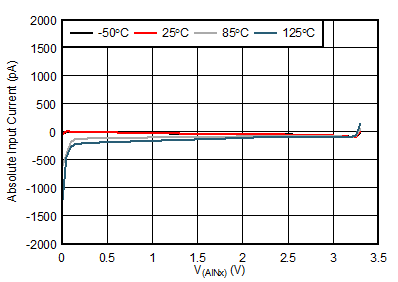PGA enabled, gain = 1, DR = 20 SPS, VIN = 0 V
Figure 10. Absolute Input Current vs Absolute Input Voltage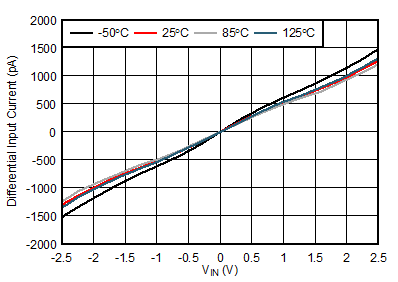PGA bypassed, DR = 20 SPS, VCM = 1.65 V
Figure 12. Differential Input Current vs Differential Input Voltage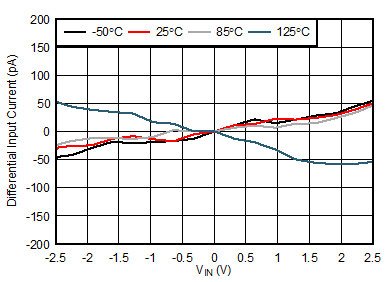PGA enabled, DR = 20 SPS, VCM = 1.65 V
Figure 14. Differential Input Current vs Differential Input Voltage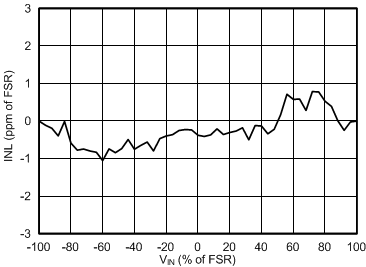PGA bypassed, gain = 1
Figure 16. INL vs Differential Input Voltage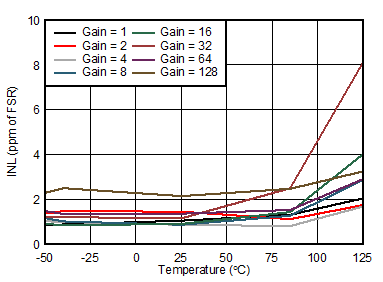Figure 18. INL vs Temperature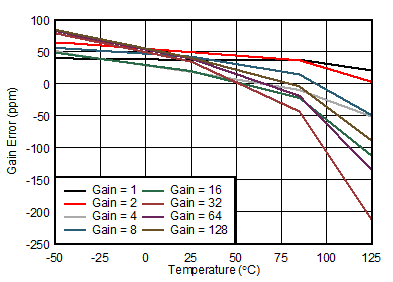Figure 20. Gain Error vs Temperature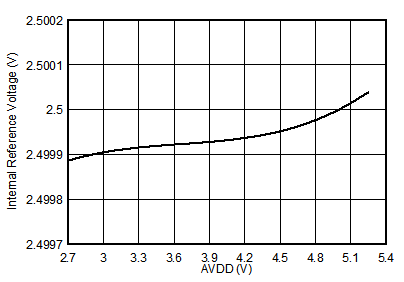Figure 22. Internal Reference Voltage vs AVDD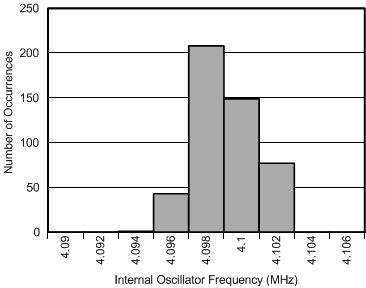Figure 24. Internal Oscillator Frequency Histogram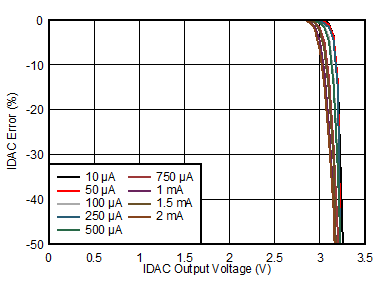Figure 26. IDAC Accuracy vs Compliance VoltageIDAC output voltage = 1.65 V
Figure 28. IDAC Accuracy vs Temperature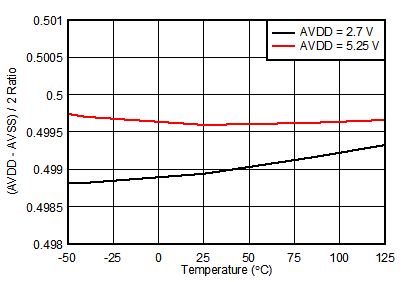Figure 30. VBIAS Voltage [(AVDD – AVSS) / 2] vs Temperature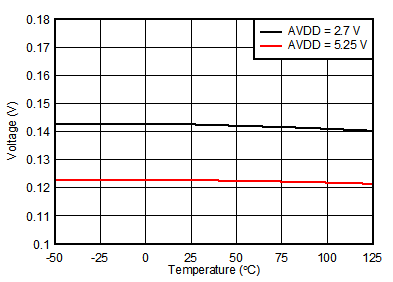Figure 32. PGA Rail Detection, PGAN_RAILP, PGAP_RAILP Threshold From AVDD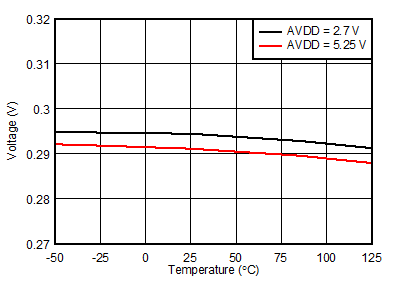Level 0 = 300 mV
Figure 34. Reference Threshold Voltage, Level 0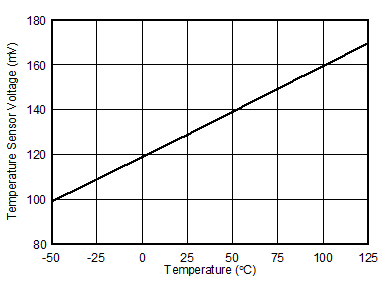Figure 36. Temperature Sensor Voltage vs Temperature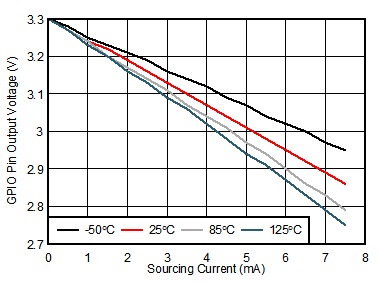AVDD = 3.3 V
Figure 38. GPIO Pin Output Voltage vs Sourcing Current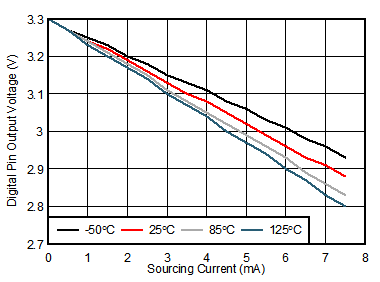DVDD = 3.3 V
Figure 40. Digital Pin Output Voltage vs Sourcing Current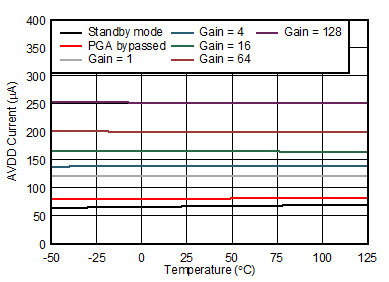Standby and conversion mode, external VREF
Figure 42. Analog Supply Current vs Temperature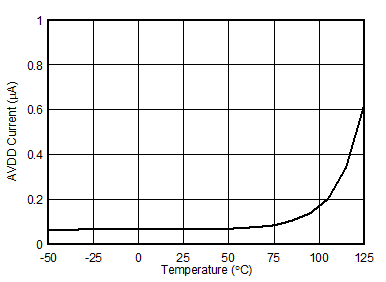Power-down mode
Figure 44. Analog Supply Current vs Temperature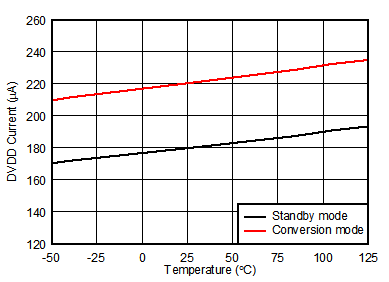Standby and conversion mode
Figure 46. Digital Supply Current vs Temperature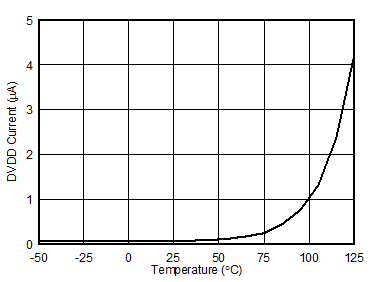Power-down mode
Figure 48. Digital Supply Current vs Temperature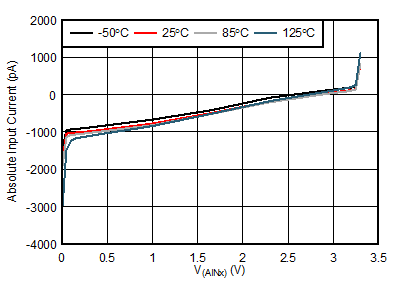PGA bypassed, DR = 4 kSPS, VIN = 0 V
Figure 9. Absolute Input Current vs Absolute Input Voltage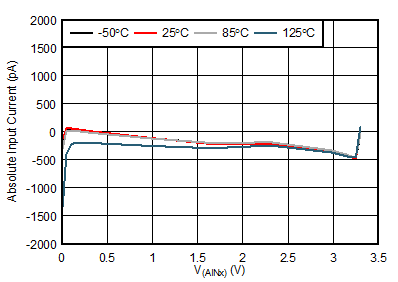PGA enabled, gain = 1, DR = 4 kSPS, VIN = 0 V
Figure 11. Absolute Input Current vs Absolute Input VoltagePGA bypassed, DR = 4 kSPS, VCM = 1.65 V
Figure 13. Differential Input Current vs Differential Input VoltagePGA enabled, DR = 4 kSPS, VCM = 1.65 V
Figure 15. Differential Input Current vs Differential Input Voltage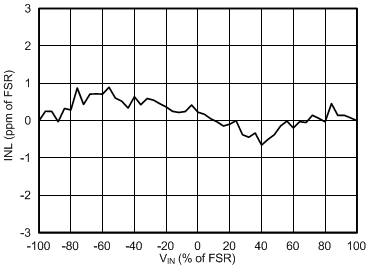PGA enabled, gain = 1
Figure 17. INL vs Differential Input Voltage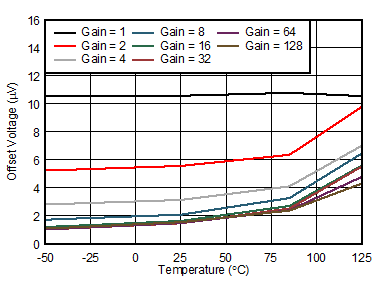Figure 19. Offset Voltage vs Temperature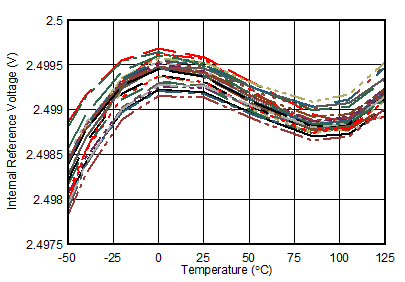28 units, TQFP package
Figure 21. Internal Reference Voltage vs Temperature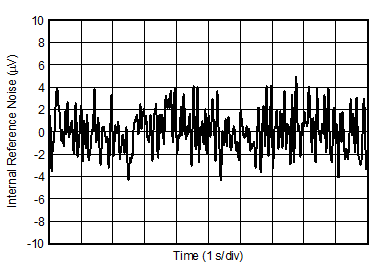Figure 23. Internal Reference Voltage Noise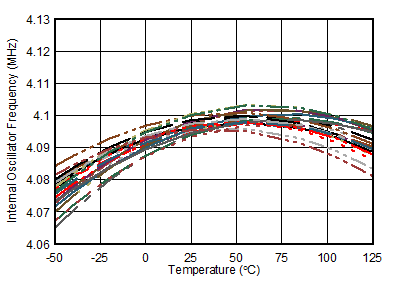28 units
Figure 25. Internal Oscillator Frequency vs Temperature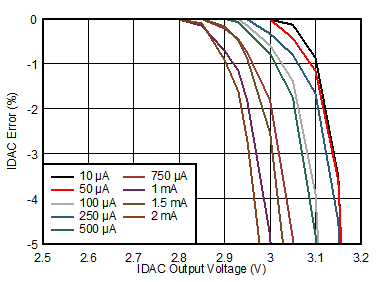Figure 27. IDAC Accuracy vs Compliance Voltage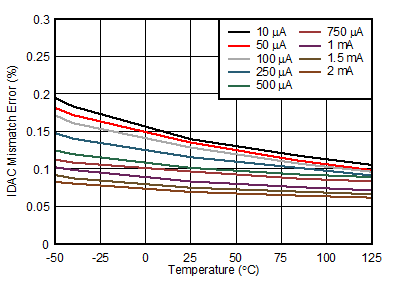Figure 29. IDAC Matching vs Temperature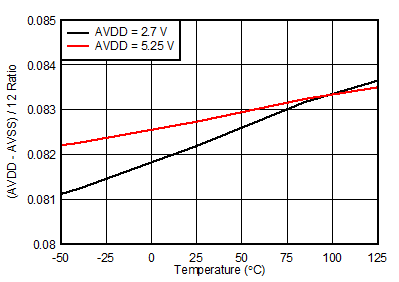Figure 31. VBIAS Voltage [(AVDD – AVSS) / 12] vs Temperature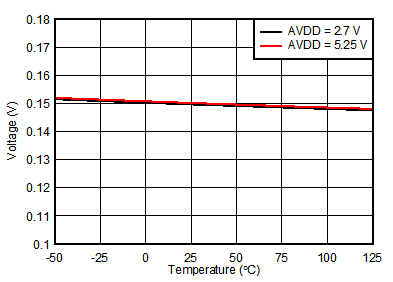Figure 33. PGA Rail Detection, PGAN_RAILN, PGAP_RAILN Threshold From AVSS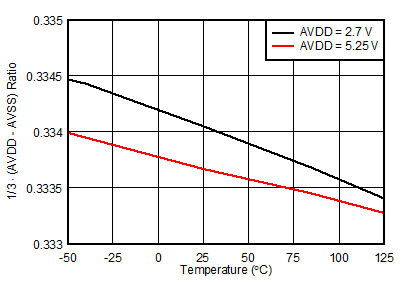Level 1 = 1/3 · (AVDD – AVSS)
Figure 35. Reference Threshold Voltage, Level 1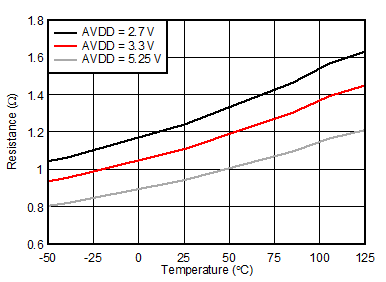Figure 37. Low-Side Switch RON vs TemperatureAVDD = 3.3 V
Figure 39. GPIO Pin Output Voltage vs Sinking CurrentDVDD = 3.3 V
Figure 41. Digital Pin Output Voltage vs Sinking Current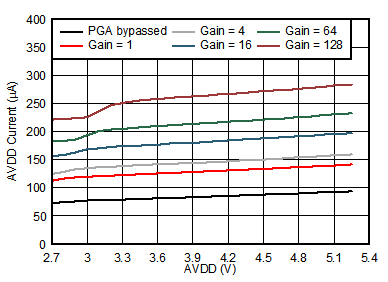Conversion mode, external VREF
Figure 43. Analog Supply Current vs AVDD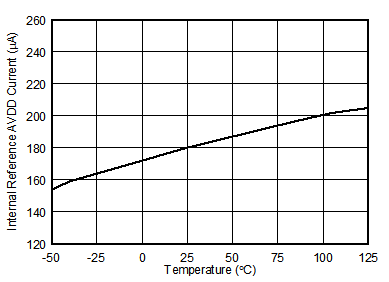Figure 45. Internal Reference AVDD Current vs TemperatureConversion mode
Figure 47. Digital Supply Current vs DVDD